# How to Calculate Activity Based Costing

Activity Based Costing (ABC) is a costing approach, that allocates manufacturing overheads into per unit cost in a more rational manner compared to the traditional costing approaches. This activity based costing method is known as a two-step approach where the overhead expenses are allocated to the respective activities known as cost pools first and those costs are assigned to the product thereafter. Different costs will be assigned only for products that the same activity is demanded, creating a more accurate and logical cost per unit value. This article explains how to calculate activity based costing in this two step approach.

Activity Based Costing focuses on the activities. It operates under the assumption that, different activities occur all the overhead costs and different products use such activities at different amounts. Thus, it expects to acquire a logical cost per unit in the end.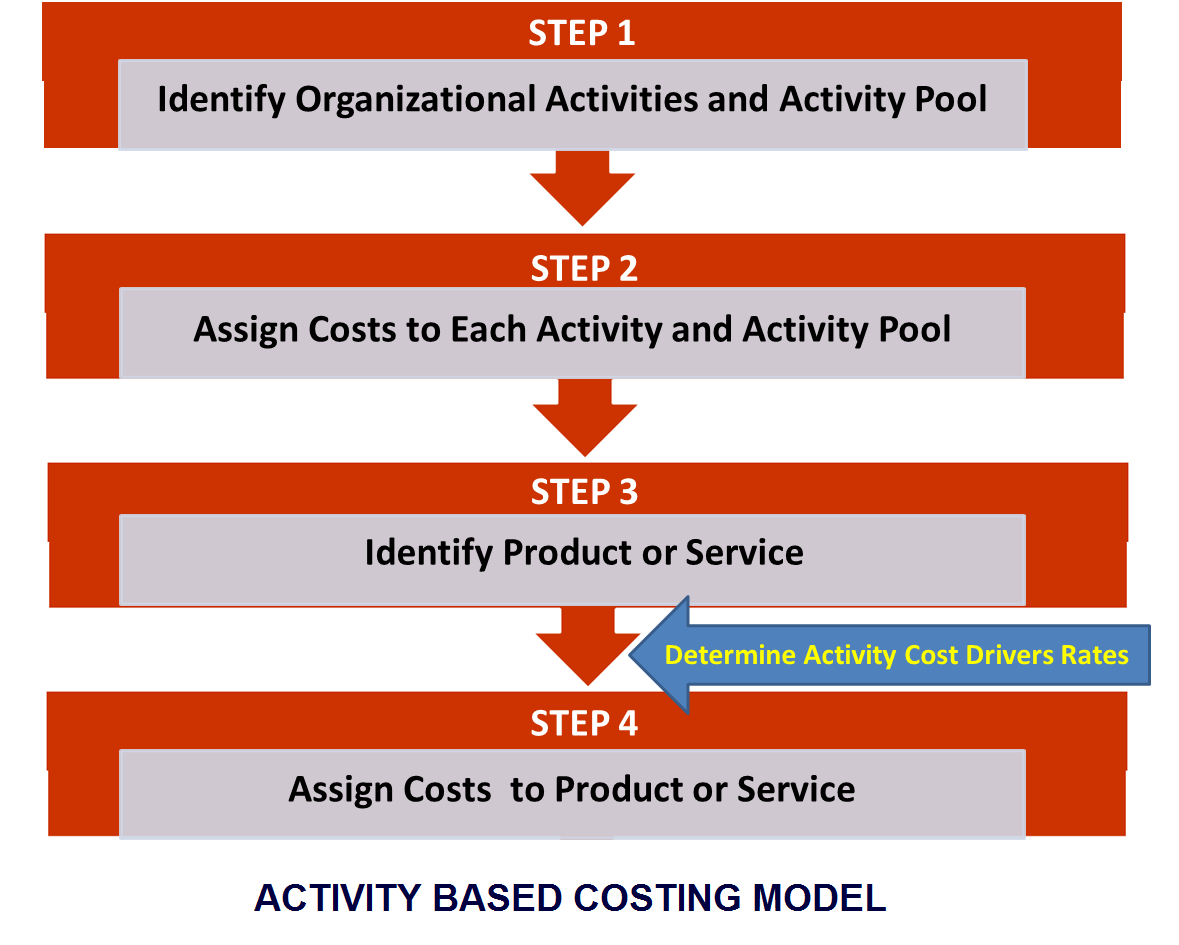## Calculate Activity Based Costing – Example

Delta Ltd is a manufacturer of two types of electrical tools known as Specialty and Standard. Product Standard sells for \$ 36 per unit and Specialty sells for \$ 40 per unit. The following information is given regarding the production volume, direct material and direct labour costs.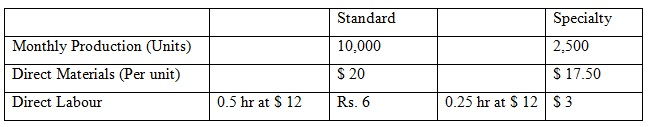Initially, overhead was absorbed to the product on a traditional approach. However, the newly appointed Management Accountant is keen on introducing activity based costing system to calculate the cost per unit.

Following information has been gathered.

• Estimated total overhead cost was \$ 101,250
• Estimated direct labour hours were \$ 5,625

Following information relates to different activities and its costs for Delta Ltd.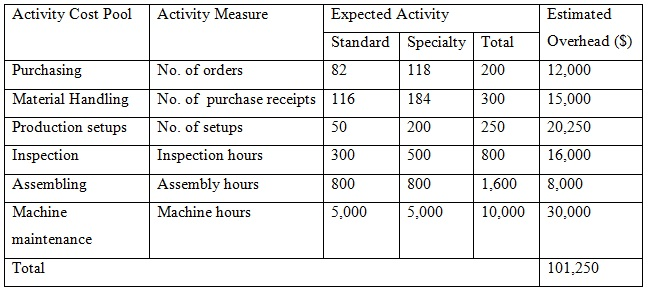Based on the information obtained, cost per unit for the two products can be calculated using account based costing method as,

Step 1:

Calculate the overhead rates for each activity.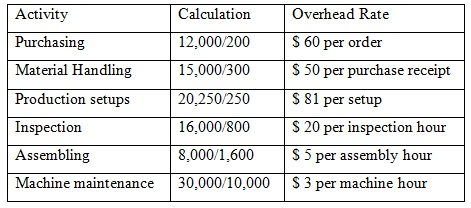Step 2:

Allocation of cost of each activity to two products.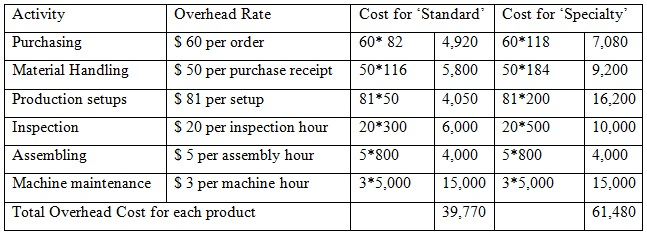Step 3:

Allocation the of total cost into production units.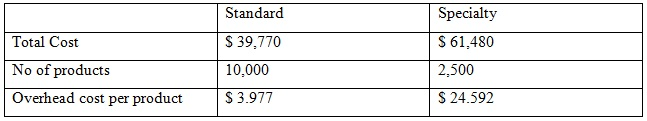Step 4:

Calculating Cost per Unit.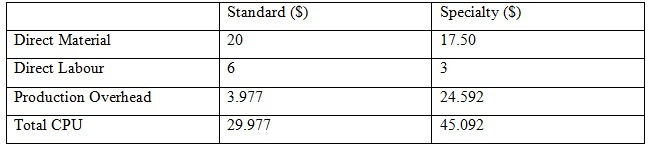Since Delta Ltd has initially calculated its overhead costs based on the traditional method, it is worthwhile to calculate the cost per unit  for the same two products using the traditional method in order to facilitate better comparison.

Total overhead cost = \$ 101,250
No. of direct labour hour:
Standrd – 0.5 hrs per unit * 10,000 units = 5,000 hs
Speciaty’– 0.25 hrs per unit * 2,500 units = 625 hrs
Total no. of hours (5,000 + 625) = 5,626 hrs

Then, a common overhead rate can be calculated taking the base as direct labour hours. So,

\$ 101,250/5.625 = \$ 18 per labour hour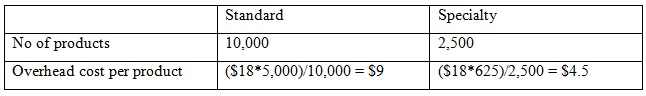Therefore, cost per unit can be calculated as follows.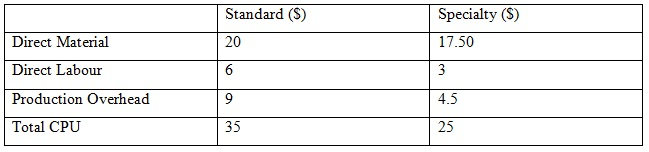Comparison cost per unit value between two different methods can be illustrated as follows.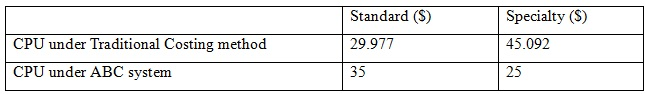Therefore, it is clear that account based costing system is more rational compared to the traditional costing approach, since it considers the utilization of each of the activities by the products rather than mere going for a common basis. The pricing has also been affected s Delta Ltd has used traditional costing system initial.